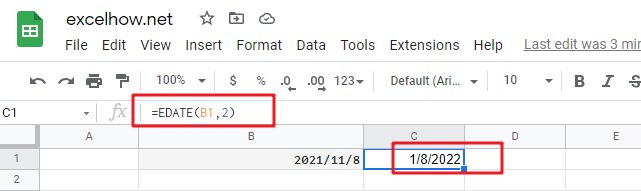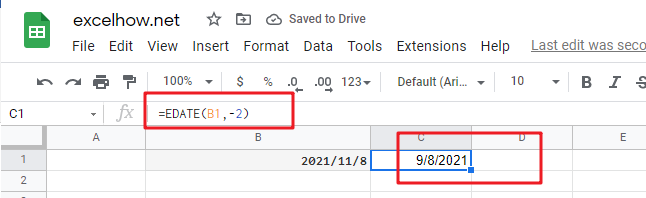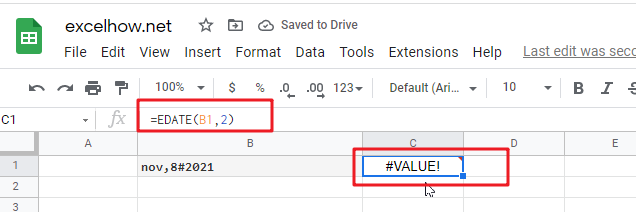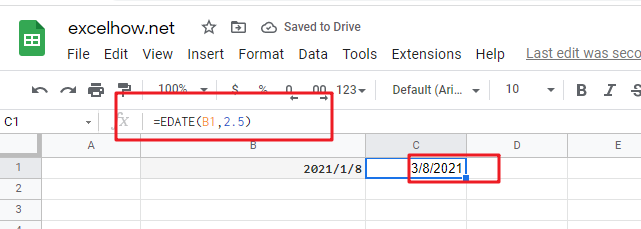# ExcelHow

This post will guide you how to use Google Sheets EDATE function with syntax and examples.

## Description

The Google Sheets EDATE returns a date that is a specified number of months before or after a specified date. You can use the EDATE function to calculate the expiration dates or maturity dates or due dates that fall on the same day of the month as the date of issue. or you can add a specified number of months to a date.

The EDATE function can be used to get a date a specified number of months before or after date in google sheets. The purpose of this function is to shift date a specified months in future or post and its returned values is a new date.

The EDATE function is a build-in function in Google Sheets and it is categorized as a Date function.

## Syntax

The syntax of the EDATE function is as below:

=EDATE (start_date, months)

Where the EDATE function arguments are:

• Start_date -This is a required argument. Adate that represents the start date.
• months – This is a required argument. the number of months before or after start_date. if you provide a positive number for months, it represents the future date; if it is a negative number, then it represents a past date.

Notes:

• google sheets stores dates as a serial number, it indicated the number of days since January 1,1900. and the date January 1,1900 is serial number 1.
• if `start_date` is not a valid date, the EDATE function will return the #VALUE! error.
• if months is not an integer, it is truncated.
• If the `start_date` has a fractional time component, it will be removed.
• If the `start_date` argument contains a decimal value, it will be removed.

## Google Sheets EDATE Function Examples

The below examples will show you how to use google sheets EDATE Function to return the serial number.
1# set a positive number for months argument in the EDATE function

`=EDATE(B1,2)`2# set a negative number for months argument in the EDATE function, using the following formula:

`=EDATE(B2,-2)`3# set a invalid date for start_date argument in the EDATE function, type the following formula:

`=EDATE(B1,2)`4# set a value that is not an integer for months arguments, using the following formula:

`=EDATE(B4,2)`